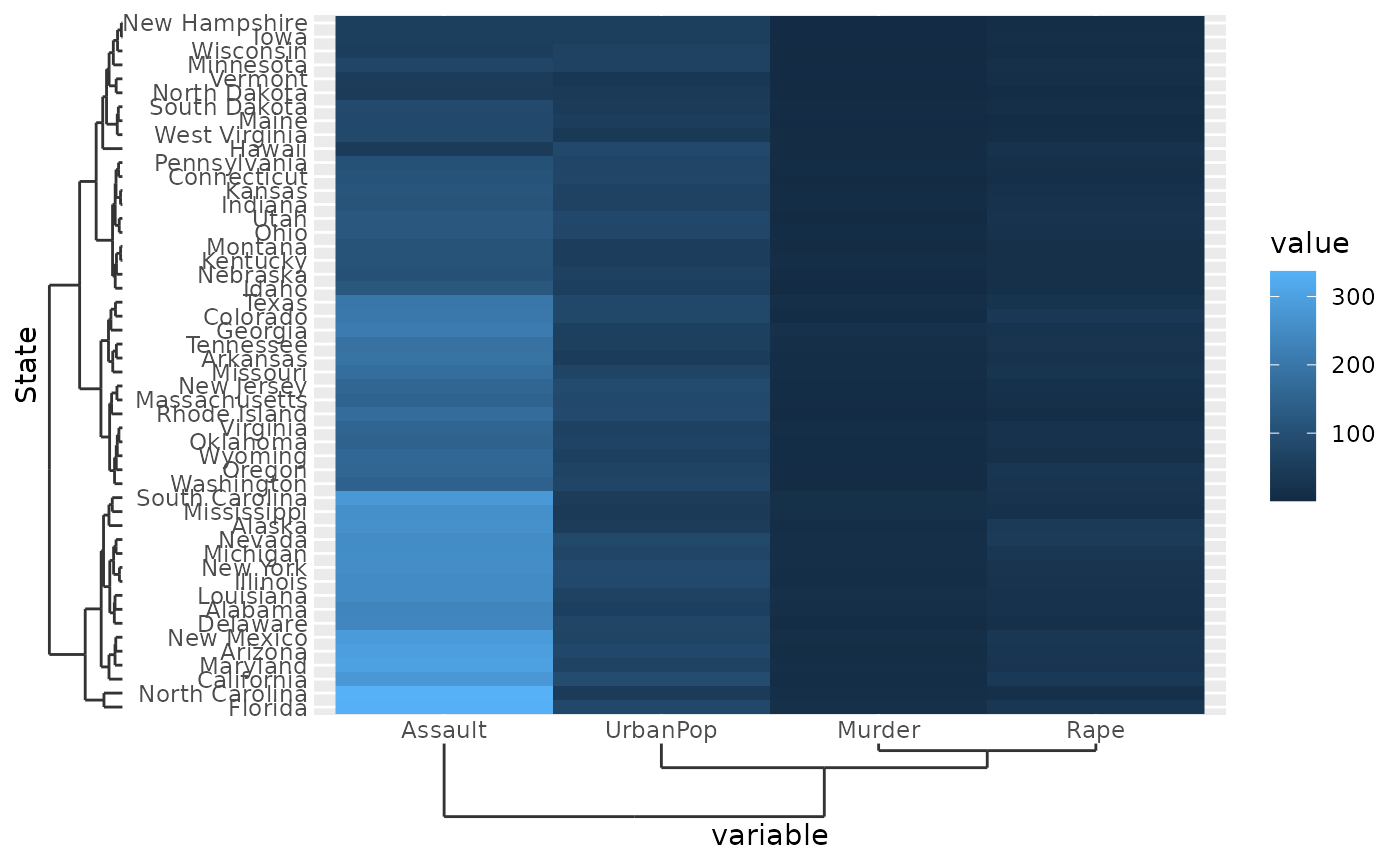When discrete data has some inherent hierarchy to the relationship between discrete categories, you can display a dendrogram instead of a tick axis.

## Usage

scale_x_dendrogram(
...,
hclust = waiver(),
expand = waiver(),
guide = waiver(),
position = "bottom"
)

scale_y_dendrogram(
...,
hclust = waiver(),
expand = waiver(),
guide = waiver(),
position = "left"
)

## Arguments

...

Arguments passed on to ggplot2::discrete_scale

aesthetics

The names of the aesthetics that this scale works with.

scale_name

The name of the scale that should be used for error messages associated with this scale.

palette

A palette function that when called with a single integer argument (the number of levels in the scale) returns the values that they should take (e.g., scales::hue_pal()).

name

The name of the scale. Used as the axis or legend title. If waiver(), the default, the name of the scale is taken from the first mapping used for that aesthetic. If NULL, the legend title will be omitted.

labels

One of:

• NULL for no labels

• waiver() for the default labels computed by the transformation object

• A character vector giving labels (must be same length as breaks)

• An expression vector (must be the same length as breaks). See ?plotmath for details.

• A function that takes the breaks as input and returns labels as output. Also accepts rlang lambda function notation.

limits

One of:

• NULL to use the default scale values

• A character vector that defines possible values of the scale and their order

• A function that accepts the existing (automatic) values and returns new ones. Also accepts rlang lambda function notation.

na.translate

Unlike continuous scales, discrete scales can easily show missing values, and do so by default. If you want to remove missing values from a discrete scale, specify na.translate = FALSE.

na.value

If na.translate = TRUE, what aesthetic value should the missing values be displayed as? Does not apply to position scales where NA is always placed at the far right.

drop

Should unused factor levels be omitted from the scale? The default, TRUE, uses the levels that appear in the data; FALSE uses all the levels in the factor.

super

The super class to use for the constructed scale

hclust

An object of the type produced by the stats::hclust() function.

expand

For position scales, a vector of range expansion constants used to add some padding around the data to ensure that they are placed some distance away from the axes. Use the convenience function expansion() to generate the values for the expand argument. The defaults are to expand the scale by 5% on each side for continuous variables, and by 0.6 units on each side for discrete variables.

guide

A function used to create a guide or its name. See guides() for more information.

position

For position scales, The position of the axis. left or right for y axes, top or bottom for x axes.

## Value

A ScaleDendrogram ggproto object.

## Details

The dendrogram type of scale does two things, first it reorders the values along the relevant direction such that they follow the order captured in the hclust argument. Secondly, it draws the dendrogram at the axis. The dendrogram visuals inherit from the ticks theme elements, so defining a linetype for the tick marks sets the linetype for the dendrogram.

## Examples

# Hierarchically cluster USArrests
yclus <- hclust(dist(USArrests), "ave")
xclus <- hclust(dist(t(USArrests)), "ave")

# Melting USArrests
df <- data.frame(
State = rownames(USArrests)[row(USArrests)],
variable = colnames(USArrests)[col(USArrests)],
value = unname(do.call(c, USArrests))
)

# Supply the clustering to the scales
ggplot(df, aes(variable, State, fill = value)) +
geom_raster() +
scale_y_dendrogram(hclust = yclus) +
scale_x_dendrogram(hclust = xclus)